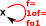# Maths - Category Theory - Comparing Objects

When comparing objects it is often more interesting when two sets are not identical but preserve some form of structure when mapping between them.relative strength type and category notation description
tightest Equality C and D are the same in these terms
Isomorphism
1d = GF
FG = 1c
There must be an arrow and inverse between the objects and when composed these give the identity map for every element.
Equivalence
1dGF
FG1cFor equivalence there is a arrow in both directions between the two objects. It is not necessary that GF and FG are the identity elements but they must be isomorphic to the identity elements.

loosestunit:
μ : 1c =>GF
co-unit:
ξ : FG => 1d

 triangle identities: as 2-cells:For adjunctions there is a arrow in both directions between the two objects. It is not necessary that GF and FG are the identity elements but only that they have natural transformations to the identity elements.

FG is unit (does not change object - injective followed by surjective) but GF does change object (surjective followed by injective). Like equivalence but in one direction.

Alternative definition: there is an isomorphism:
φ: D(FA , X) -> C(A, GX)
for every AC and XD

## Equivalence Relations

In a less category specific approach to binary relations they may, or may not, have the following properties:

Equivalence Relation Group
xytransitive
 xy yz
xzreflexive xxsymmetric
 xy yx## Comparison of Sets

Here we investigate this comparisons with the simplest concrete categories - sets.

type of
comparison

Sets GF
EqualityIsomorphism
1d = GF
FG = 1cEquivalence
1dGF
FG1cWhere I can, I have put links to Amazon for books that are relevant to the subject, click on the appropriate country flag to get more details of the book or to buy it from them.The Princeton Companion to Mathematics - This is a big book that attempts to give a wide overview of the whole of mathematics, inevitably there are many things missing, but it gives a good insight into the history, concepts, branches, theorems and wider perspective of mathematics. It is well written and, if you are interested in maths, this is the type of book where you can open a page at random and find something interesting to read. To some extent it can be used as a reference book, although it doesn't have tables of formula for trig functions and so on, but where it is most useful is when you want to read about various topics to find out which topics are interesting and relevant to you.